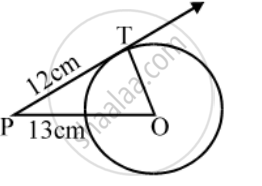# A point P is 13 cm from the centre of the circle. The length of the tangent drawn from P to the circle is 12cm. Find the radius of the circle. - Mathematics

Sum

A point P is 13 cm from the centre of the circle. The length of the tangent drawn from P to the circle is 12cm. Find the radius of the circle.

#### Solution

Since tangent to a circle is perpendicular to the radius through the point of contact.∴ ∠OTP = 90°

In right triangle OTP, we have

OP^2 = OT^2 + P^2

⇒ 13^2 = OT^2 + 12^2

⇒ OT^2 = 13^2 – 12^2

= (13 – 12) (13 + 12) = 25

⇒ OT = 5

Concept: Concept of Circle - Centre, Radius, Diameter, Arc, Sector, Chord, Segment, Semicircle, Circumference, Interior and Exterior, Concentric Circles
Is there an error in this question or solution?
Share